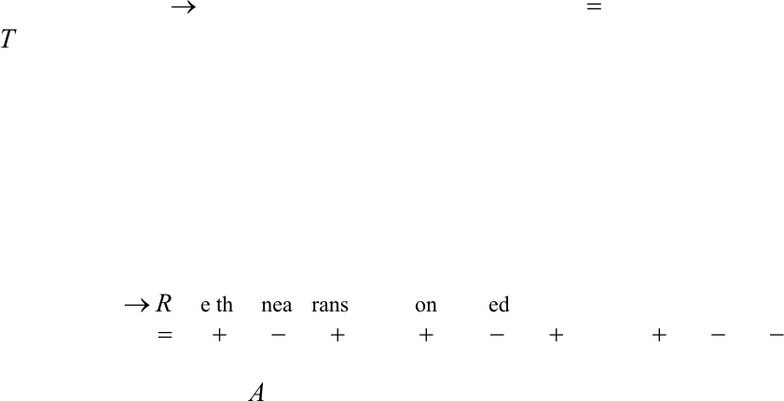Get 2 days of premium access
Study Guides (380,000)
US (220,000)
LSU (10,000)
MATH (400)
All (10)

# Tesreview3Exam

Department
Mathematics
Course Code
MATH 2090
Professor
All

This preview shows page 1. to view the full 4 pages of the document.Testreview3. Review sections: 5.1, 5.3, 5.6, 5.7, 6.1-6.3, and 6.7. (Review also HW
assignments)
1. Let
2
: ( )T M R R
be the transformation defined by
( ) det( )T A A=
. Show that
T
is a linear transformation or show, by example, which property for linear
transformations fails.
2. Let
43
:T R R
be the linear transformation defined by
1 2 3 4 1 2 3 4 1 2 3 4 1 2 3 4
( , , , ) ( 3 2 ,3 10 4 6 ,2 5 6 )T x x x x x x x x x x x x x x x x= + + + + +
(a) Find the matrix,
A
, for T;
(b) Find a basis for the
()Ker T
and its dimension;
###### You're Reading a Preview

Unlock to view full version